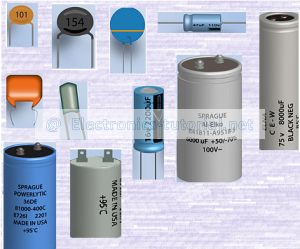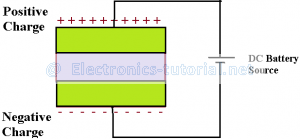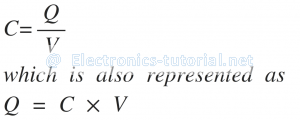Home > electronic components > capacitors > capacitorbasics

# Capacitor basics

Capacitor is a kind of passive component which can be named as a condenser. The basic function of capacitor is to store the energy in the form of electric charge. Inside capacitor there are two plates which are separated by the dielectric material. On these two plates the charge is stored n the capacitor which creates the potential difference between the two plates . Hence, capacitor seems like a battery which stores the electricity. The different types of capacitors are available in the market all of them do the same task of energy storage.

The capacitor consists of more than two metallic plates which are separated by the insulating material e.g. plastic, mica, ceramic etc. This kind of insulating material is called as dielectric material. Because of this insulating material between the metal plates the direct current i.e. DC supply is blocked and forms image electric charge across the plates of the capacitor.

The metal plates of the capacitor can have any shape i.e parallel rectangle, cylindrical shape and circular shape depending upon the application of capacitor in the different voltages. The typical shape of the capacitor is shown in the figure below.In order to use the capacitor in the DC networks consisting of resistor(R), inductor (L), and capacitor (C) shortly called as RLC circui, the capacitor is charged upto the battery voltage applied. However, the capacitor do not allow the flow of current between the two metallic plates. This is because the metallic plates of the capacitor are separated by the dielectric material. Now instead of DC battery if we connect the source of alternating current i.e. AC current, then the current will flow through the capacitor and in this case capacitor will act as a short circuit which is having minimum resistance.

As we know that, the battery is having two polarities i.e one in negative (-) polarity charge and the other is positive (+) polarity charge. When the battery having DC storage is connected to the capacitor then at one metallic plate of the capacitor the '+' charge is accumulated and due to the principle of image force charge the image of â€œ- â€œ charge is created at the other plate of the battery. E.g. if 10 â€œ+â€ charges are accumulated at one plate then the correspongly 10 â€œâ€“â€œ charges are accumulated at the other plate of the capacitor.

Therefore, in this manner the charges are stored in the parallel plate capacitor. Depending upon the charge storage capacity i.e. capacitance value the capacitor reaches the steady state value and beyond that capacitor can not store the extra charge and the current through the capacitor is stop flowing. This is decided by the parallel plates and the dielectric material present between the two plates of the capacitor.

The current flowing through the capacitor at during the charging of the is popularly known as the charging current. The charging current through the capacitor is continusly flowing unless and until the â€œ+â€ charge at one plate is exactly equal to the â€œ-â€œ charge at the other plate. Once flow charging current is stopped that condition is known as the capacitor is fully charged condition. When the capacitor is fully charged then now it can work as a charged battry. Now hen the capacitor is connected in the DC circuit then it ctrts discharging and the current start flowing through the capacitor. This current is called as discharging current. When the charge at both the parallel plates is removed completely then the discharge current stops flowing and this condition is called as the capacitor is fully discharged. In this situation there is no charge at the plates of the capacitor. Hence, there is no existence of the potential difference between the plates of the capacitor. The potential difference on the plates of the capacitor is mainly due to the amount of electric charge stored on the plates of the capacitor. This storage is known as the storage capacity of the capacitor.The formation of image charges in capacitor are shown in the figure below.The simplest form of capacitor is the parallel plate capacitor where two metallic plates are separated by the dielectric material. The capacitance value of this capacitor is decided by the cross-sectional area of the metallic plates, the dielectric constant of the dielectric material and the distance between the two metallic plates. By changing the value of any of these parameters the capacitance value of the capacitor changes. Since capacitor stores the electrical energy in the form of electron charge on the plate, the area of plate is the important parameter. Larger the ara of metallic plate, larger is the storage capacity of the capacitor. Also, the distance between the metallic plates also decides the storage capacity of the capacitor. Smaller is the distance between the two metallic plates larger is the storage capacity of the capacitor.

When we apply the potential â€œVâ€ to the capacitor through the battery the charge â€œQâ€ will be stored into the capacitor. Therefore, the capacitance of the capacitor which is measured in Farad and given by,As we know that, the electric field and electric potential are related to each other. The electric field due to the DC potential is called as the electrostatic field. Hence the potential difference between the two metallic plates in the capacitor stores the energy in the form of electrostatic field. Hence, when the charging of the capacitor is started due to the flow of the charging current, the electrostatic field around the metallic plates of the capacitor increases. On the other hand, when discharging of the capacitor starts i.e. discharge current removes the static charge stored on the plates of the capacitor the electrostatic field around the plates of the capacitor reduces. This clearly indicates that, the pf the capacitor s to store the electrostatic fields on the plates of the capacitor.
The measuring unit of capacitor is Farad which is nothing but the capacity of storing 1 Coulomb charge by the application of 1 Volt potential through the battery. The symbol of capacitance is â€œCâ€. The graphical symbol of capacitor is as shown in figure below,Capacitance Units:Capacitors Basics